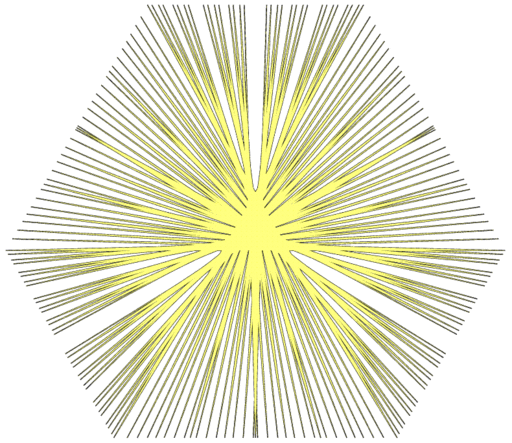### Highlights#### Waves, Kakeya sets, and Diophantine equations

MSRI - June 2017Ciprian Demeter

A central problem in Physics is to understand the complicated ways in which waves can interact with one another. The field of Harmonic Analysis grew out of the observation that any natural signal that is periodic in time can be built by superimposing simple waves with whole number frequencies. In geometric optics, quantum mechanics, and the study of water waves, we must understand the interactions of waves in space, where waves have not just a frequency and an amplitude, but also a direction. In the late 1960s, a systematic study of the interactions of spatial waves led to the development of Restriction Theory, which is concerned with the interactions of waves whose frequencies and directions are highly constrained. This investigation has revealed deep and surprising connections with a mysterious class of geometric objects, known as “Kakeya sets.” A Kakeya set is a collection of length one line segments (we think of needles having thickness zero) that point in all possible directions. The simplest Kakeya set is the interior of a circle of radius 1 (the unit disk), since for each direction, there is a corresponding line segment joining the center to the boundary of the circle. The disk has positive area and can easily be drawn and visualized, but there are examples of Kakeya sets that are invisible not only to the human eye, but to arbitrarily large orders of magnification. Even stranger, there exist sets of arbitrarily small area such that one may continuously rotate a needle through all possible directions, while staying inside the set.

The “Kakeya Set Conjecture” predicts that Kakeya sets become visible under a certain very special mathematical microscope called Hausdorff dimension. While this problem has only been fully solved in two spatial dimensions, attempts to solve it in higher dimensions have led to spectacular developments on related problems. As an example, approximately ten years ago, a simplified, discrete, model for the Kakeya Set Conjecture was solved. This version has connections with theoretical computer science, and the tool developed to solve it involves careful counting arguments using polynomials. A few years later, the same method was expanded to count the minimum number of distances determined by a fixed number of points in the plane. More recently, the polynomial method has been finely tuned to estimate more efficiently the cancellations between different waves. The idea is that we can use a relatively small collection of relatively simple polynomials to divide space into small cells, in such a way that the wave interactions inside each cell are easier to understand. The implementation of this approach required the development of connections between areas of mathematics that were hitherto thought to be unrelated.

In the last three years we have discovered a new method, called “decoupling,” to quantitatively study wave interactions. Once again, the connection with the Kakeya phenomenon proved instrumental. This discovery was initially motivated by the study of the Schrödinger equation, which models the energy of quantum mechanical systems, but decouplings proved to have a host of other far reaching consequences in an unexpected area of mathematics: Diophantine equations. Diophantine equations are potentially complicated systems of equations involving whole numbers, and mathematicians are interested in counting the number of solutions to such systems. Unlike waves, numbers do not oscillate, at least not in an obvious manner, but we can think of numbers as frequencies, and thus associate them to waves. In this way, problems related to counting the number of solutions to Diophantine systems can be rephrased in the language of quantifying wave interferences. An example of a Diophantine problem where the decoupling approach has been useful is in counting the number of ways a given whole number can be written as a sum of perfect cubes; another involves subtle issues around the intricate behavior of prime numbers. It is expected that the decoupling phenomenon will find new applications in the future, and the Spring 2017 MSRI program has articulated a few new exciting directions.